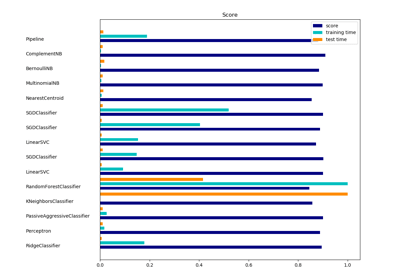/scikit-learn

sklearn.linear_model.RidgeClassifier

class sklearn.linear_model.RidgeClassifier(alpha=1.0, fit_intercept=True, normalize=False, copy_X=True, max_iter=None, tol=0.001, class_weight=None, solver=’auto’, random_state=None) [source]

Classifier using Ridge regression.

Read more in the User Guide.

Parameters: alpha : float Regularization strength; must be a positive float. Regularization improves the conditioning of the problem and reduces the variance of the estimates. Larger values specify stronger regularization. Alpha corresponds to C^-1 in other linear models such as LogisticRegression or LinearSVC. fit_intercept : boolean Whether to calculate the intercept for this model. If set to false, no intercept will be used in calculations (e.g. data is expected to be already centered). normalize : boolean, optional, default False This parameter is ignored when fit_intercept is set to False. If True, the regressors X will be normalized before regression by subtracting the mean and dividing by the l2-norm. If you wish to standardize, please use sklearn.preprocessing.StandardScaler before calling fit on an estimator with normalize=False. copy_X : boolean, optional, default True If True, X will be copied; else, it may be overwritten. max_iter : int, optional Maximum number of iterations for conjugate gradient solver. The default value is determined by scipy.sparse.linalg. tol : float Precision of the solution. class_weight : dict or ‘balanced’, optional Weights associated with classes in the form {class_label: weight}. If not given, all classes are supposed to have weight one. The “balanced” mode uses the values of y to automatically adjust weights inversely proportional to class frequencies in the input data as n_samples / (n_classes * np.bincount(y)) solver : {‘auto’, ‘svd’, ‘cholesky’, ‘lsqr’, ‘sparse_cg’, ‘sag’, ‘saga’} Solver to use in the computational routines: ‘auto’ chooses the solver automatically based on the type of data. ‘svd’ uses a Singular Value Decomposition of X to compute the Ridge coefficients. More stable for singular matrices than ‘cholesky’. ‘cholesky’ uses the standard scipy.linalg.solve function to obtain a closed-form solution. ‘sparse_cg’ uses the conjugate gradient solver as found in scipy.sparse.linalg.cg. As an iterative algorithm, this solver is more appropriate than ‘cholesky’ for large-scale data (possibility to set tol and max_iter). ‘lsqr’ uses the dedicated regularized least-squares routine scipy.sparse.linalg.lsqr. It is the fastest and uses an iterative procedure. ‘sag’ uses a Stochastic Average Gradient descent, and ‘saga’ uses its unbiased and more flexible version named SAGA. Both methods use an iterative procedure, and are often faster than other solvers when both n_samples and n_features are large. Note that ‘sag’ and ‘saga’ fast convergence is only guaranteed on features with approximately the same scale. You can preprocess the data with a scaler from sklearn.preprocessing. New in version 0.17: Stochastic Average Gradient descent solver. New in version 0.19: SAGA solver. random_state : int, RandomState instance or None, optional, default None The seed of the pseudo random number generator to use when shuffling the data. If int, random_state is the seed used by the random number generator; If RandomState instance, random_state is the random number generator; If None, the random number generator is the RandomState instance used by np.random. Used when solver == ‘sag’. coef_ : array, shape (n_features,) or (n_classes, n_features) Weight vector(s). intercept_ : float | array, shape = (n_targets,) Independent term in decision function. Set to 0.0 if fit_intercept = False. n_iter_ : array or None, shape (n_targets,) Actual number of iterations for each target. Available only for sag and lsqr solvers. Other solvers will return None.

Ridge
Ridge regression
RidgeClassifierCV
Ridge classifier with built-in cross validation

Notes

For multi-class classification, n_class classifiers are trained in a one-versus-all approach. Concretely, this is implemented by taking advantage of the multi-variate response support in Ridge.

Examples

>>> from sklearn.datasets import load_breast_cancer
>>> from sklearn.linear_model import RidgeClassifier
>>> X, y = load_breast_cancer(return_X_y=True)
>>> clf = RidgeClassifier().fit(X, y)
>>> clf.score(X, y)
0.9595...

Methods

 decision_function(X) Predict confidence scores for samples. fit(X, y[, sample_weight]) Fit Ridge regression model. get_params([deep]) Get parameters for this estimator. predict(X) Predict class labels for samples in X. score(X, y[, sample_weight]) Returns the mean accuracy on the given test data and labels. set_params(**params) Set the parameters of this estimator.
__init__(alpha=1.0, fit_intercept=True, normalize=False, copy_X=True, max_iter=None, tol=0.001, class_weight=None, solver=’auto’, random_state=None) [source]
decision_function(X) [source]

Predict confidence scores for samples.

The confidence score for a sample is the signed distance of that sample to the hyperplane.

Parameters: X : array_like or sparse matrix, shape (n_samples, n_features) Samples. array, shape=(n_samples,) if n_classes == 2 else (n_samples, n_classes) Confidence scores per (sample, class) combination. In the binary case, confidence score for self.classes_ where >0 means this class would be predicted.
fit(X, y, sample_weight=None) [source]

Fit Ridge regression model.

Parameters: X : {array-like, sparse matrix}, shape = [n_samples,n_features] Training data y : array-like, shape = [n_samples] Target values sample_weight : float or numpy array of shape (n_samples,) Sample weight. New in version 0.17: sample_weight support to Classifier. self : returns an instance of self.
get_params(deep=True) [source]

Get parameters for this estimator.

Parameters: deep : boolean, optional If True, will return the parameters for this estimator and contained subobjects that are estimators. params : mapping of string to any Parameter names mapped to their values.
predict(X) [source]

Predict class labels for samples in X.

Parameters: X : array_like or sparse matrix, shape (n_samples, n_features) Samples. C : array, shape [n_samples] Predicted class label per sample.
score(X, y, sample_weight=None) [source]

Returns the mean accuracy on the given test data and labels.

In multi-label classification, this is the subset accuracy which is a harsh metric since you require for each sample that each label set be correctly predicted.

Parameters: X : array-like, shape = (n_samples, n_features) Test samples. y : array-like, shape = (n_samples) or (n_samples, n_outputs) True labels for X. sample_weight : array-like, shape = [n_samples], optional Sample weights. score : float Mean accuracy of self.predict(X) wrt. y.
set_params(**params) [source]

Set the parameters of this estimator.

The method works on simple estimators as well as on nested objects (such as pipelines). The latter have parameters of the form <component>__<parameter> so that it’s possible to update each component of a nested object.

Returns: self

Examples using sklearn.linear_model.RidgeClassifierClassification of text documents using sparse features

© 2007–2018 The scikit-learn developers